Categories

# What are we learning?

We will learn to find tangent plane by solving an IIT JAM 2018 Problem. This is the Question no. 5 of the IIT JAM 2018 Solved Paper Series. Go through this link for Question no. 6. Gradient is one of the key concepts of vector calculus. We will use this problem from IIT JAM 2018 to clear our concepts.

# Understand the problem

The tangent plane to the surface$z= \sqrt{x^2+3y^2}$ at (1,1,2) is given by
1. $x-3y+z=0$
2. $x+3y-2z=0$
3. $2x+4y-3z=0$
4. $3x-7y+2z=0$
IIT Jam 2018
Easy
##### Suggested Book
 Calculus: Multi-Variable Calculus and Linear Algebra with Applications to Differential Equations and Probability – Vol 2 Tom M. Apostol

# Look at the knowledge graph…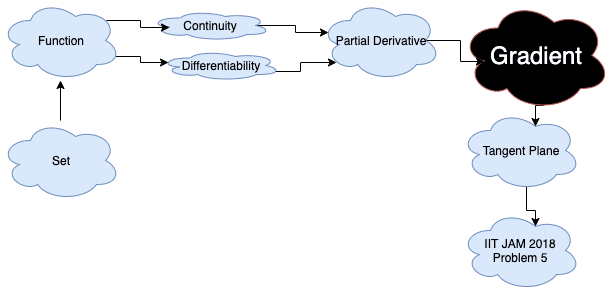Do you really need a hint? Try it first!

Given a differentiable function $Z=f(x,y)$, Observe that when we are asked to find a tangent plane at $(x_0,y_0,z_0)$ then the picture that comes in our mind is a plane that touches the curve at a point.

When we are in dimension $2$ it is just a line, (easy to visualize), dim 3 a plane (still visible), dim 4,5,…. a surface which is hard to see, but we can plug in $x=x_0$ in the equation $z=f(x,y)$ to have $z=f(x_0,y)$ which is just a curve in 2D then we can visualize the tangent line at $y=y_0$ is a part of the tangent plane $z=f(x,y)$ isn’t it?? The same thing is true of about the tangent line at $x=x_0$ for the curve $z=f(x,y_0)$. These $f(x,y_0)$ and $f(x_0,y)$ are called sections of the curve $f(x,y)=z$ . Here $(x_0,y_0,z_0)=(1,2,3)$. So, quickly find out $f(1,y)$ and $f(x,1)$.

You can see that $f(1,y)=\sqrt{1+3y^{2}}$ and $f(x,1)= \sqrt{x^{2}+3}$ Now observe that the tangent plane of the curve $z=f(x,y)$ is a plane right !! What will be the basic structure of a plane at $(x_0,y_0,z_0)$?

It is a $a(x-x_0)+ b(y-y_0)+ c(z-z_0)=0$ ———————–(1) Now see that $(x_0,y_0,z_0)=(1,1,2)$ is already given in the question. Hence the unknown is $(a,b,c)$ . Equation (1) implies $z = z_0+ \frac{a}{c}(x-x_0)+ \frac{b}{c}(y-y_0)$ Differentiating the equation by $x$ we get, $z_x= \frac{a}{c}$ Differentiating the equation by $y$ we get, $z_y= \frac{b}{c}$ Hence the equation of the tangent plane is $z=z_0+z_x|_{(x_0,y_0)}(x-x_0)+ z_y|_{(x_0,y_0)}(y-y_0)$ So calculate $z_x$ and $z_y$ at $(x_0,y_0)$

$z_x = \frac{d}{dx}f(x,1)= \frac{2x}{2\sqrt{x^{2}+3}}|_{(1,1)} = \frac{2}{4}= \frac{1}{2}$ $z_y=\frac{d}{dy}f(1,y)=\frac{6y}{2\sqrt{1+3y^2}}|_{(1,1)}=\frac{6}{2 \times 2}=\frac{3}{2}$ So the equation of the tangent line is $z= 2+\frac{1}{2}(x-1)+\frac{3}{2}(y-1)$ $\Rightarrow 2z= 4+x-1+3y-3$

$x+3y-2z=0$ (Ans)

[h5p id="5"]

# Play with graph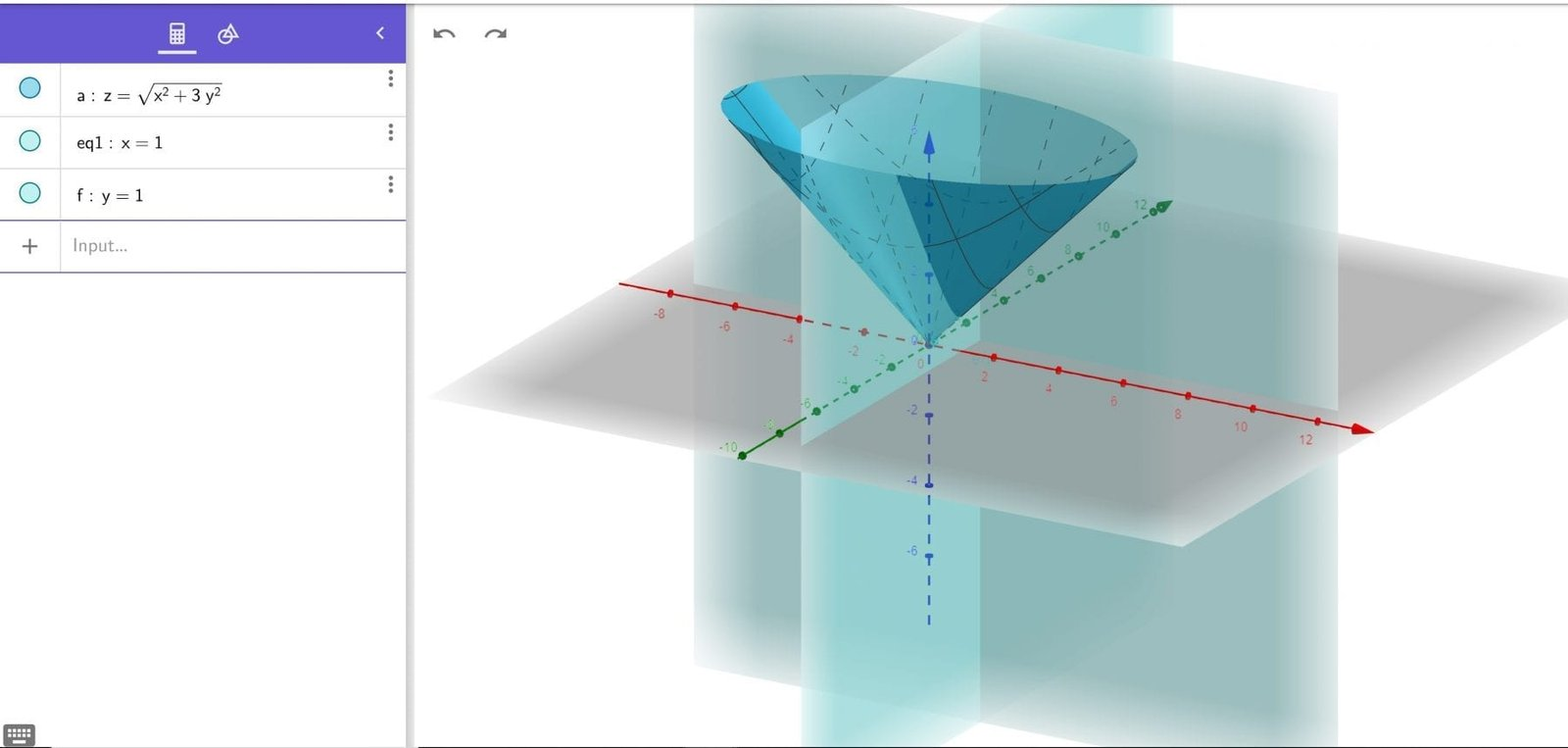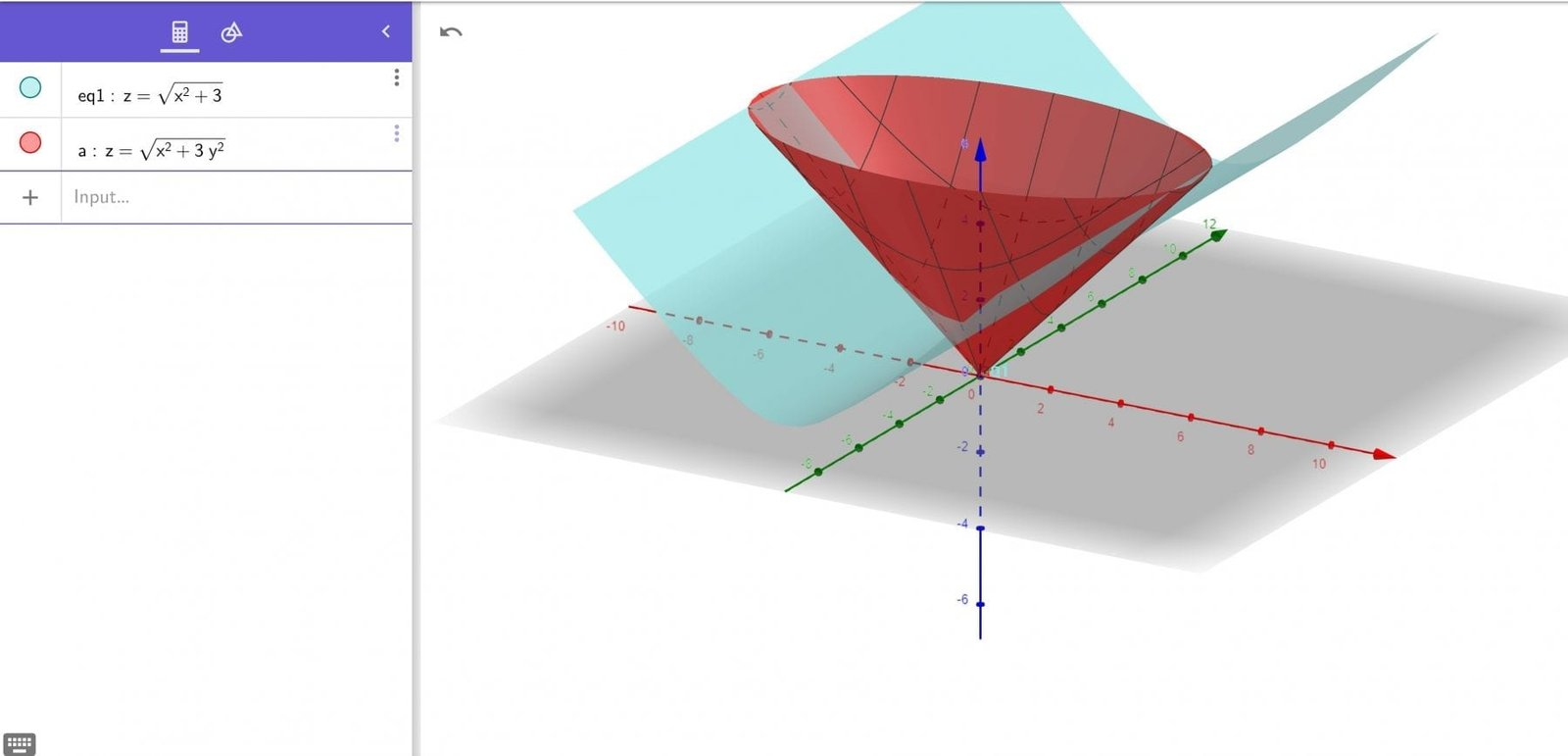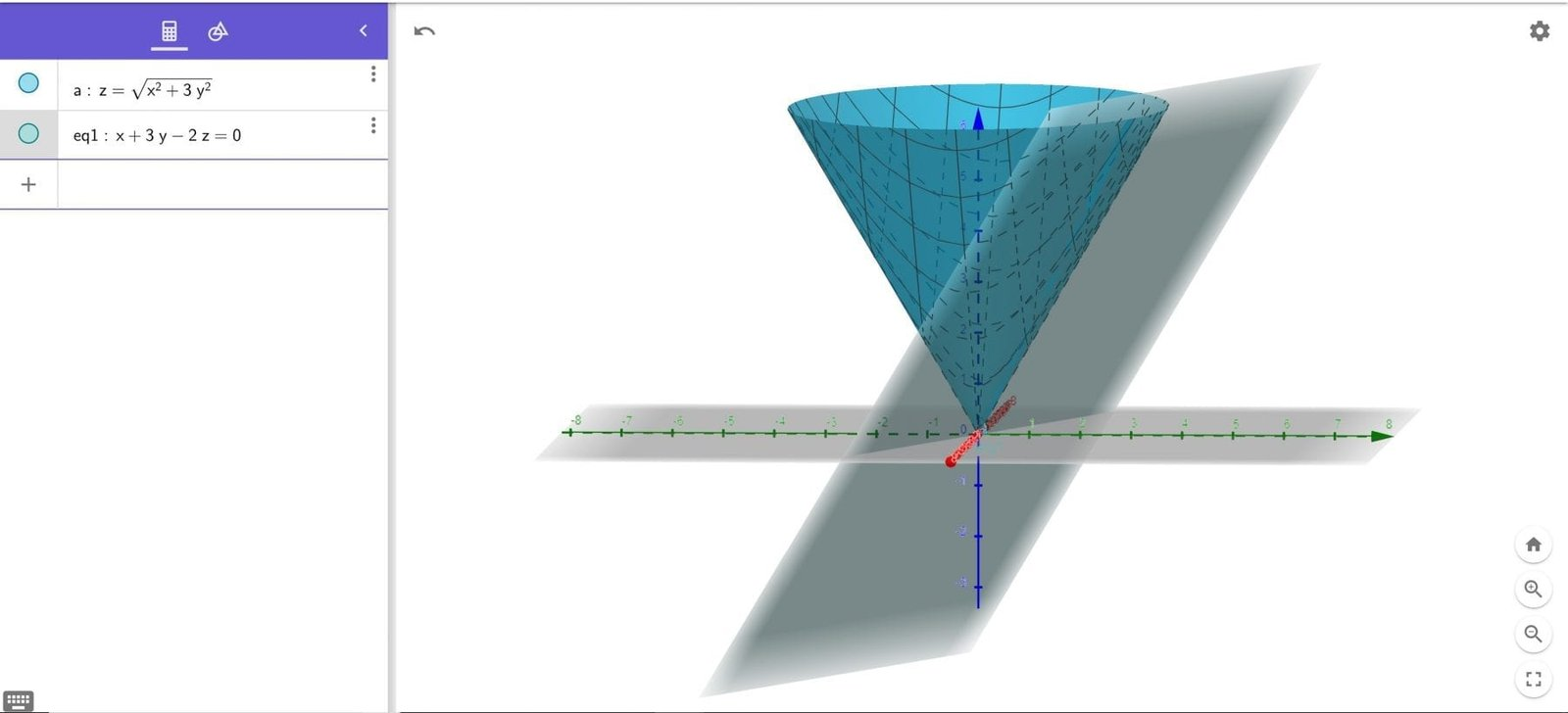# Connected Program at Cheenta

#### College Mathematics Program

The higher mathematics program caters to advanced college and university students. It is useful for I.S.I. M.Math Entrance, GRE Math Subject Test, TIFR Ph.D. Entrance, I.I.T. JAM. The program is problem driven. We work with candidates who have a deep love for mathematics. This program is also useful for adults continuing who wish to rediscover the world of mathematics.

# Similar Problems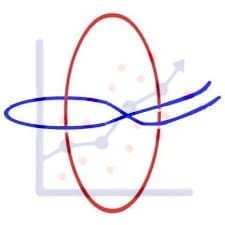## ISI MStat PSB 2006 Problem 8 | Bernoullian Beauty

This is a very simple and regular sample problem from ISI MStat PSB 2009 Problem 8. It It is based on testing the nature of the mean of Exponential distribution. Give it a Try it !## ISI MStat PSB 2009 Problem 8 | How big is the Mean?

This is a very simple and regular sample problem from ISI MStat PSB 2009 Problem 8. It It is based on testing the nature of the mean of Exponential distribution. Give it a Try it !## ISI MStat PSB 2009 Problem 4 | Polarized to Normal

This is a very beautiful sample problem from ISI MStat PSB 2009 Problem 4. It is based on the idea of Polar Transformations, but need a good deal of observation o realize that. Give it a Try it !## ISI MStat PSB 2009 Problem 6 | abNormal MLE of Normal

This is a very beautiful sample problem from ISI MStat PSB 2009 Problem 6. It is based on the idea of Restricted Maximum Likelihood Estimators, and Mean Squared Errors. Give it a Try it !## ISI MStat PSB 2009 Problem 3 | Gamma is not abNormal

This is a very simple but beautiful sample problem from ISI MStat PSB 2009 Problem 3. It is based on recognizing density function and then using CLT. Try it !## ISI MStat PSB 2009 Problem 1 | Nilpotent Matrices

This is a very simple sample problem from ISI MStat PSB 2009 Problem 1. It is based on basic properties of Nilpotent Matrices and Skew-symmetric Matrices. Try it !## ISI MStat PSB 2006 Problem 2 | Cauchy & Schwarz come to rescue

This is a very subtle sample problem from ISI MStat PSB 2006 Problem 2. After seeing this problem, one may think of using Lagrange Multipliers, but one can just find easier and beautiful way, if one is really keen to find one. Can you!

## Problem on Inequality | ISI – MSQMS – B, 2018 | Problem 2a

Try this problem from ISI MSQMS 2018 which involves the concept of Inequality. You can use the sequential hints provided to solve the problem.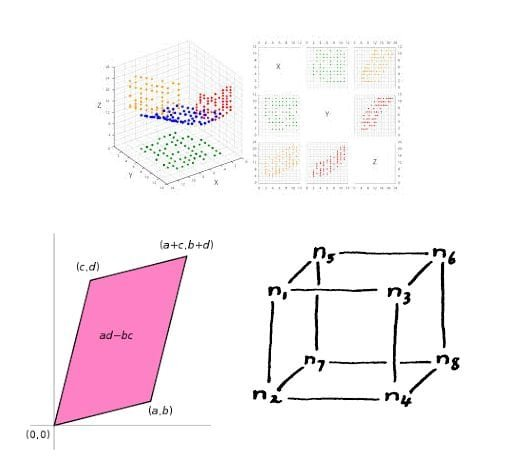## Data, Determinant and Simplex

This problem is a beautiful problem connecting linear algebra, geometry and data. Go ahead and dwelve into the glorious connection.

## Problem on Integral Inequality | ISI – MSQMS – B, 2015

Try this problem from ISI MSQMS 2015 which involves the concept of Integral Inequality and real analysis. You can use the sequential hints provided to solve the problem.

This site uses Akismet to reduce spam. Learn how your comment data is processed.